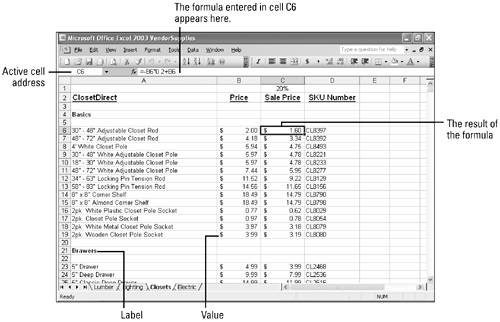# Making Label Entries

 There are three basic types of cell entries: labels, values, and formulas. A label is text in a cell that identifies the data on the worksheet so readers can interpret the information. Excel does not use labels in its calculations. For example, the label price is used as a column header to identify the price of each item in the column. A value is a number you enter in a cell. Excel knows to include values in its calculations. To enter values easily and quickly, you can format a cell, a range of cells , or a column with a specific number- related format. Then, as you type, the cells are formatted automatically. To perform a calculation in a worksheet, you enter a formula in a cell. A formula is a calculation that contains cell references, values, and arithmetic operators. The result of a formula appears in the worksheet cell where you entered the formula. The contents of the cell appears on the formula bar. Entering cell references rather than actual values in a formula has distinct advantages. When you change the data in the worksheet or copy the formula to other cells (copying this formula to the cell below), Excel automatically adjusts the cell references in the formula and returns the correct results.Show Me Microsoft Office Excel 2003
ISBN: 0789730057
EAN: 2147483647
Year: 2002
Pages: 291